# Summary findings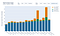Figure 1. Transit heavy congested cities are most at risk of travel time spikes. Travel times are given as one-way commute times. On the interactive plot, switch sets of cities and sort order with the buttons on top, and mouse over bars to see details. See the interactive version of this plot at https://lab-work.github.io/therebound/.

# Data analysis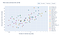Figure 2. Across cities, average travel times increase with more vehicles on the road. See the interactive version of this plot at https://lab-work.github.io/therebound/.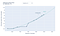Figure 3. Within each city, we see how adding cars increases travel times. See the interactive version of this plot at https://lab-work.github.io/therebound/.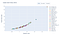Figure 4. Travel time ratios increase in a predictable way as the capacity ratio increases. This is true even across cities. See the interactive version of this plot at https://lab-work.github.io/therebound/.

# Traffic scenarios during the rebound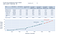Figure 5: Travel time predictions if transit users switch to cars. See the interactive version of this plot at https://lab-work.github.io/therebound/.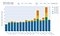Figure 6: Some of the largest one-way travel time increases are observed for cities with many transit riders. See the interactive version of this plot at https://lab-work.github.io/therebound/.

# Research Team

Research scientist at Vanderbilt University working in transportation systems, artificial intelligence, and data science.

## More from Will Barbour

Research scientist at Vanderbilt University working in transportation systems, artificial intelligence, and data science.